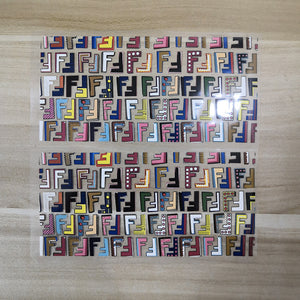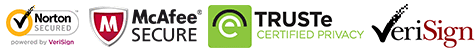FedEx will stop during Feb 8-18, due to the new year holidy, Free shipping still works
FedEx will stop during Feb 8-18, due to the new year holidy, Free shipping still works# Colorful Fendi Patches for Custom Air Force 1 Fendi, Iron on Fendi Logo Patches For Shoes Decal

Regular price \$15.00 \$9.90 Save \$5.10

ONLY 519579 LEFT

 Hours   Minutes   Seconds

Sale Ends Once The Timer Hits Zero!

ONLY 519579 LEFT

Get these easy use iron on colorful Fendi patches to create your own Fendi shoes. Best size for DIY or custom  Air Force 1 & Vans.

<<<<<<<<<<<<<<<<<<<<<<<<<<<<<<<<<<<<<<<<<<<<<<<<<<<<<<<

Please STOP wasting money to buy the "NO RETURN, NO REFUND Fendi shoes. Try this iron on Fendi stickers to DIY. Save lots of money!

<<<<<<<<<<<<<<<<<<<<<<<<<<<<<<<<<<<<<<<<<<<<<<<<<<<<<<<

Why use these colorful Fendi patches:

• 1. Perfect sizes for sneakers and other items, such as socks, hoodies, jeans, jackets....
• 2. Waterproof and flexible, when iron on, proved durable after washing test and brushing test. You can check the videos at the bottom of front page or my YouTube Channel: www.youtube.com/c/theshoesgirl
• 3. Easy use, just iron on directly, no need any transfer paper, no need any sealer. Will not peel off even brushing in water! You can watch the test in my videos.
• 4. Durable but still can be removed, if you are tired with this design after several months, remove them and change another design to refresh your shoes.

<<<<<<<<<<<<<<<<<<<<<<<<<<<<<<<<<<<<<<<<<<<<<<<<<<<<<<<

• ### The set includes:

• 2 sheets of colorful Fendi patches
<<<<<<<<<<<<<<<<<<<<<<<<<<<<<<<<<<<<<<<<<<<<<<<<<<<<<<<
• ### Size:

#### Fendi sheet:

width: 16.5 cm; height: 8 cm

<<<<<<<<<<<<<<<<<<<<<<<<<<<<<<<<<<<<<<<<<<<<<<<<<<<<<<<

### How to use:

1. Position the fendi patches on the shoes with tape, mark on the tape, then cut along with the marks.

2. Iron on the rest part of the patches, please keep temperature 135-150℃

3. peel the vinyl, the rest of fendi patches will stick on the shoes.

4. If use normal iron, please turn off the steam, keep the temperature above, use the tip and be careful and patient, for it's heavy to control, better use the mini iron.

5. Watch the tutorial video here:

https://www.youtube.com/watch?v=jTH5lnoB4Xo

<<<<<<<<<<<<<<<<<<<<<<<<<<<<<<<<<<<<<<<<<<<<<<<<<<<<<<<

FREE SHIPPING! Ship within 1-2 days.

people are currently looking at this product

Estimated delivery time 7-15 days

Guaranteed Safe Checkout